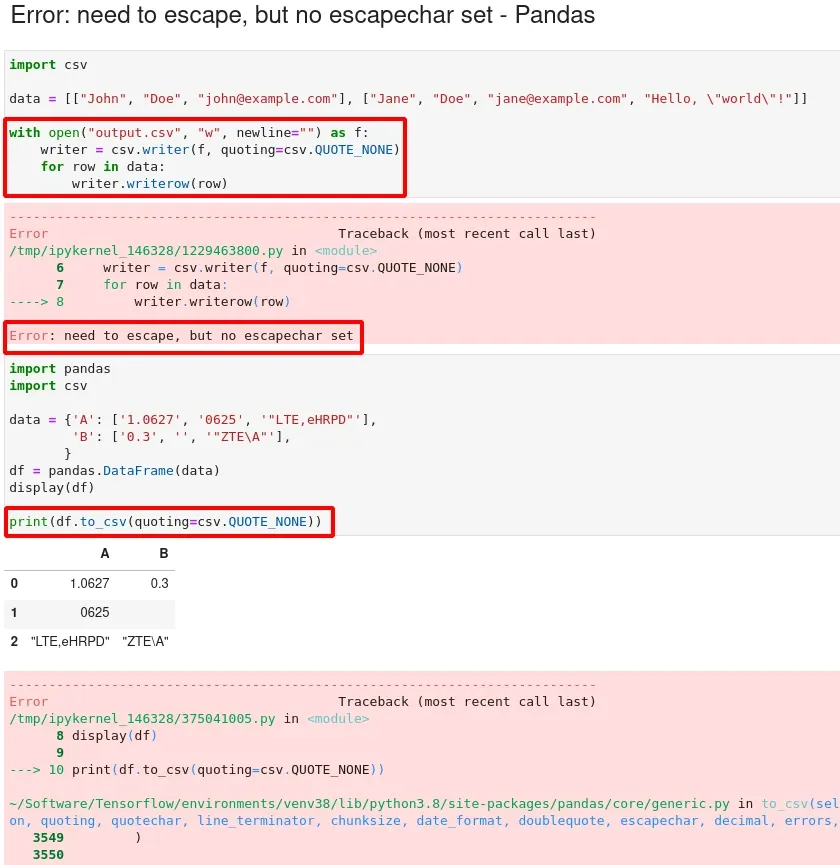In this tutorial, we'll see how to solve a common Pandas and Python error – "Error: need to escape, but no escapechar set". We get this error from Pandas when we try to save DataFrame as a CSV file.

Let's see several examples of how to reproduce and solve this error.

## Pandas - Error: need to escape, but no escapechar set

When we try to use `to_csv` we may get error in Pandas:

Error: need to escape, but no escapechar set

The example below demonstrate the error:

``````import pandas
import csv

data = {'A': ['1.0627', '0625', '"LTE,eHRPD"'],
'B': ['0.3', '', '"ZTE\A"'],
}
df = pandas.DataFrame(data)
display(df)

print(df.to_csv(quoting=csv.QUOTE_NONE))
``````

The error is caused by using `csv.QUOTE_NONE`. Let's check two different solutions to this error.

## Pandas to_csv escapechar

First way to solve the error is by using `escapechar='\\'`:

``````print(df.to_csv(quoting=csv.QUOTE_NONE, quotechar='',escapechar='\\'))
``````

This will produce CSV file as:

``````,A,B
0,1.0627,0.3
1,0625,
2,"LTE\,eHRPD","ZTE\\A"
``````

## Solution - QUOTE_ALL and QUOTE_MINIMAL

Alternatively we can solve the error by setting parameters:

• `quoting=csv.QUOTE_MINIMAL` - to only quote fields that contain special characters.
• `quoting=csv.QUOTE_ALL` - all fields will be quoted

### QUOTE_MINIMAL

We can see the output of:

``````df.to_csv(quoting=csv.QUOTE_MINIMAL)
``````

the will be quoting the values in row 2:

``````,A,B
0,1.0627,0.3
1,0625,
2,"""LTE,eHRPD""","""ZTE\A"""
``````

### QUOTE_ALL

We can see the output of - `QUOTE_ALL`:

``````df.to_csv(quoting=csv.QUOTE_ALL)
``````

then all values will be quoted:

``````"","A","B"
"0","1.0627","0.3"
"1","0625",""
"2","""LTE,eHRPD""","""ZTE\A"""
``````## Python - Error: need to escape, but no escapechar set

The code below shows how to reproduce Python error - "Error: need to escape, but no escapechar set"

### QUOTE_MINIMAL

``````import csv

data = [["John", "Doe", "[email protected]"], ["Jane", "Doe", "[email protected]", "Hello, \"world\"!"]]

with open("output.csv", "w", newline="") as f:
writer = csv.writer(f, quoting=csv.QUOTE_NONE)
for row in data:
writer.writerow(row)
``````

result:

``````Error: need to escape, but no escapechar set
``````

So the error is solved by:

``````writer = csv.writer(f, quoting=csv.QUOTE_MINIMAL)
``````

### Filter quoted values

As alternative solution we can filter the values by list comprehension:

``````import csv

data = [["John", "", "Doe"], ["Jane", " ", "Doe"]]

with open("output.csv", "w", newline="") as f:
writer = csv.writer(f)
for row in data:
row = [s if s else " " for s in row]  # filter problematic strings
writer.writerow(row)
``````

## Summary

To summarize, in this article, we've seen how to solve Python and Pandas error:

Error: need to escape, but no escapechar set

And finally you can find useful resources which will help you to solve Pandas `to_csv` output quoting issues.